Home > ACC7 > Chapter cc310 > Lesson cc310.1.3 > Problem10-46

10-46.
1. Homework Help ✎

1. Make a table for the rule y =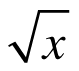that includes x‑values from −1 to 10. What happened?

2. Graph the rule on graph paper.

3. What kind of growth does the rule show? How do you know?

4. Is this relationship a function? How do you know?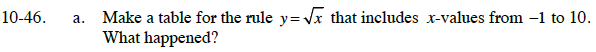What happens when you substitute a negative answer for x?
Does this make sense?

The graph has no value for x < 0.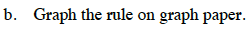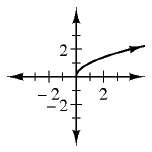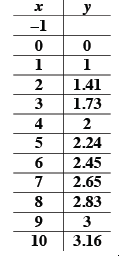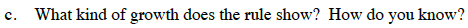Do the y-values increase by a constant amount?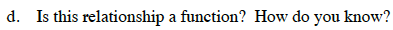Is there one output for every input?